This free and user friendly app for iPhone and iPad (it also works on Mac computers, but was not thouroughly tested and some features may not be available there) Main Features: * Draws 2D graphs of Y = F(X) functions, their derivatives and integrals * Draws stationary or animated graphs of R = F(Θ) functions in Polar coordinates * Draws stationary or animated graphs of X = F(T), Y = F(T) Parametric functions * Solves F(X,Y) = 0 equations * Draws 3D graphs of Z = F(X, Y) functions in Cartesian coordinates * Draws 3D graphs of Z = F(Θ, R) functions in Cylindrical coordinates * Draws 3D graphs of R = F(Θ, Φ) functions in Spherical coordinates Click here for more details and link to AppStore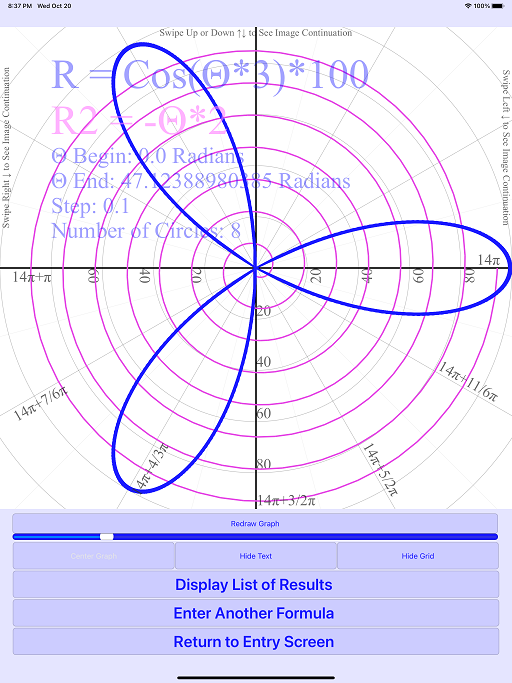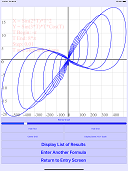y=f(x),f'(x),S(x) R=f(Θ) x=f(t),y=f(t) f(x,y)=0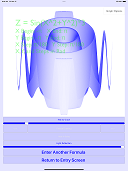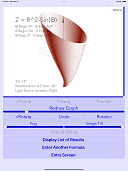z=f(x,y) z=f(Θ,R) R=f(Θ,Φ)

 This program was developed for PC with MS Windows between 2001 and 2004 Main Features: * Draws 2D graphs of Y = F(X) functions * Displays their derivatives and integrals * Draws 3D graphs of Z = F(X, Y) functions * Solves F(X,Y) = 0 equations

Here are some examples: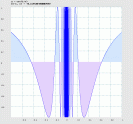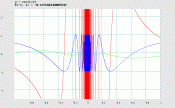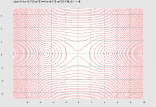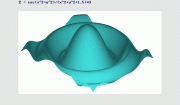y = f(x) y=f(x),f'(x),S(x) f(x,y) = 0 z = f(x,y)z = f(x,y) (rotating)

 These programs are intended for those who are learning Math and Calculus. Also, it may be helpful to engineers and researchers. For help with Algebra, Linear Algebra and Analytic Geometry, please check out our other apps, "Matrix Solver Step by Step", "Vectors and Planes", "Polynomials and Linear Systems" and "Least Squares Curve Fit" :Matrix Solver Step by Step Vectors and Planes Polynomials and Linear Systems Least Squares Curve Fit

 The authors would like to hear your suggestions or comments. Write to us! (GraphMath@aol.com)

 Keywords: graph, graphics, plot, plotting, graphing software, math, learning tools, education, 3D, three-D, 3-dimensional, tri-dimensional, function, equation, integral, derivative, extreme values, download free version, linear algebra, matrix